# Percent Timer Setting

Older pivots only have a percent timer. Use to calculate a percent timer setting to apply a desired depth or finish in a desired time period.Use the full rotation calculator to find the approximate amount of time it will take for a pivot to make a full rotation. Calculate the time for a 1" application. To see the equations used in these calculators click here. Learn more about the units used on this page.

## Percent Setting By Depth

Percent setting at known application depth (recommended to measure with catch cans):
%
Depth of water applied at the above setting:
Desired new depth to apply:
New percent timer setting to achieve desired depth:
%

## Percent Setting by Desired Completion Time

Percent timer setting at known completion time
%
Known completion time
New desired completion time:
New percent timer setting for new completion time:
%

## Percent Setting By 1" Application

Time for Full Rotation at 100%:
Application Depth Required:
Percent Timer Setting:
%

## The Equations

These calculators use these formulas to determine the percent timer setting to achieve a specified application depth or completion time.

#### Percent Setting By Depth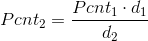Where: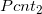= The new pivot percent timer setting to achieve desired depth (as a %)= The pivot percent timer setting to achieve known application depth (as a %)= Known application depth (in)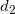= Desired new application depth (in)

#### Percent Setting By Desired Completion Time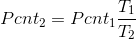Where:= The new pivot percent timer setting to achieve desired completion time (as a %)= The pivot percent timer setting to at known completion time (as a %)= Known completion time (hrs)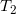= Desired completion time (hrs)

#### Percent Setting By 1" Application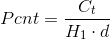Where: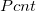= The new pivot percent setting to achieve desired depth (as a %)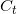= Pivot full rotation time at 100% (hrs)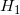= Pivot full rotation time to apply 1 inch (hrs)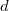= Desired application depth (in)

WSU Prosser - IAREC, 24106 N Bunn Rd, Prosser WA 99350-8694, 509-786-2226. Contact Us